### Jackknife Method

The jackknife method of variance estimation deletes one PSU at a time from the full sample to create replicates. The total number of replicates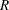is the same as the total number of PSUs. In each replicate, the sample weights of the remaining PSUs are modified by the jackknife coefficient. The modified weights are called replicate weights.

The jackknife coefficient and replicate weights are described as follows.

##### Without Stratification

If there is no stratification in the sample design (no STRATA statement), the jackknife coefficientsare the same for all replicates: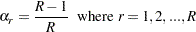Denote the original weight in the full sample for theth member of theth PSU as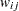. If theth PSU is included in theth replicate (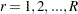), then the corresponding replicate weight for theth member of theth PSU is defined as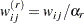##### With Stratification

If the sample design involves stratification, each stratum must have at least two PSUs to use the jackknife method.

Let stratum
be the stratum from which a PSU is deleted for theth replicate. Stratumis called the donor stratum. Let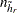be the total number of PSUs in the donor stratum. The jackknife coefficients are defined as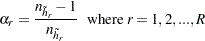Denote the original weight in the full sample for theth member of theth PSU as. If theth PSU is included in theth replicate (), then the corresponding replicate weight for theth member of theth PSU is defined as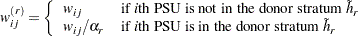You can use the VARMETHOD=JACKKNIFE(OUTJKCOEFS=) method-option to save the jackknife coefficients into a SAS data set and use the VARMETHOD=JACKKNIFE(OUTWEIGHTS=) method-option to save the replicate weights into a SAS data set.

If you provide your own replicate weights with a REPWEIGHTS statement, then you can also provide corresponding jackknife coefficients with the JKCOEFS= option.

Let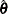be the estimated regression coefficients from the full sample for. Let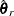be the estimated regression coefficient obtained from theth replicate by using replicate weights. PROC SURVEYLOGISTIC  estimates the covariance matrix ofby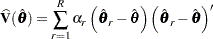with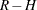degrees of freedom, whereis the number of replicates and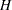is the number of strata, or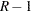when there is no stratification.